10.5 Deletion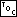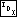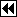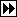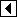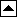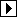Deleting a node from an RTBST can be done using the same ideas as for other kinds of trees we've seen. However, as it turns out, a variant of this usual technique allows for faster code. In this section, we will implement the usual method, then the improved version. The latter is actually used in libavl.

Here is the outline of the function. Step 2 is the only part that varies between versions:

```380. <RTBST item deletion function 380> =
void *rtbst_delete (struct rtbst_table *tree, const void *item) {
struct rtbst_node *p;	/* Node to delete. */
struct rtbst_node *q;	/* Parent of p. */
int dir;              /* Index into q->rtbst_link[] that leads to p. */

assert (tree != NULL && item != NULL);

<Step 1: Find RTBST node to delete 381>
<Step 2: Delete RTBST node, left-looking 388>
<Step 3: Finish up after deleting RTBST node 382>
}
```

This code is included in 375.

The first step just finds the node to delete. After it executes, p is the node to delete and q and dir are set such that q->rtbst_link[dir] == p.

```381. <Step 1: Find RTBST node to delete 381> =
if (tree->rtbst_root == NULL)
return NULL;

p = tree->rtbst_root;
q = (struct rtbst_node *) &tree->rtbst_root;
dir = 0;
if (p == NULL)
return NULL;

for (;;)   {
int cmp = tree->rtbst_compare (item, p->rtbst_data, tree->rtbst_param);
if (cmp == 0)
break;

dir = cmp > 0;
if (dir == 0)       {
if (p->rtbst_link == NULL)
return NULL;
}     else /* dir == 1 */       {
if (p->rtbst_rtag == RTBST_THREAD)
return NULL;
}

q = p;
}
item = p->rtbst_data;
```

This code is included in 380.

The final step is also common. We just clean up and return:

```382. <Step 3: Finish up after deleting RTBST node 382> =
tree->rtbst_alloc->libavl_free (tree->rtbst_alloc, p);
tree->rtbst_count–;
return (void *) item;
```

This code is included in 380.